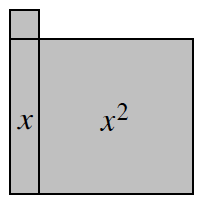### Home > CC1 > Chapter Ch6 > Lesson 6.2.5 > Problem6-115

6-115.

Sketch the algebra-tile shape at right on your paper. Write an expression for the perimeter of the shape. Then find the perimeter for each of the given values of x. Homework Help ✎

Find the perimeter as you did in problems 6-96 and 6-106, including x as part of the expression.

P = 4x + 41. $x = 7 \text{ units}$

Substitute 7 for x in the expression of the perimeter.

Evaluate the expression.
4(7) + 4 = ?

32 units

1. $x = 5.5\text{ units}$

• Substitute 5.5 for x in the expression of the perimeter and evaluate.

26 units

1. $x = \frac { 7 } { 3 }\text{ units}$

• See part (a) or (b).